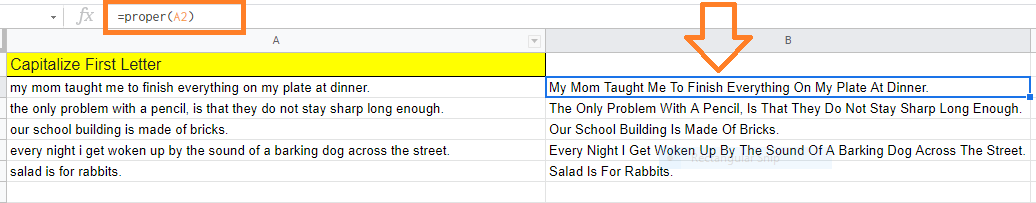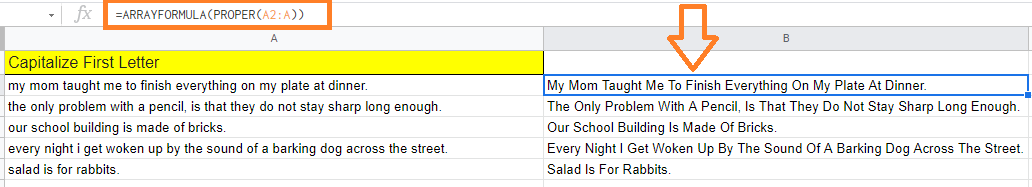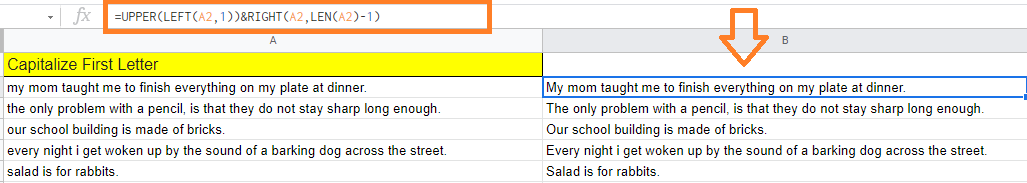# Capitalize First Letter in Google Sheets: 3 Easy Steps to Capitalise

Google Sheets comes with many text functions such as strikethrough, bold, italics, text color, and so on. Along with these functions, we also have the option to change the case of the text which is present on the Google Sheets.

In order to capitalize the first letter in Google Sheets, we will have to use the PROPER() function. However, there are other methods with the help of which we can capitalize the first letter in Google Sheets which are explained in this article.

## Capitalize First Letter of Each Word in Google Sheets

There is no inbuilt function in Google Sheets with the help of which we can capitalize the first letters in each word. With the help of the PROPER function, we can easily capitalize the first words in Google Sheets.

Let’s understand how to use the PROPER function in Google Sheets by following the steps listed below:

• Step 1: Select the cells where you would like to capitalize the first letter of each word.
• Step 2: Now enter the formula “=PROPER(A2)“.
• Step 3: Press the “Enter” button. Now you will see the results where the selected text will be capitalized.It’s easy to copy and paste the formula’s result as values once you get the desired result from it. This would ensure that the result would not change if the original dataset was edited or wiped out in the future.

Working of PROPER Function: With the help of the PROPER function, you can get the result where every word has its first letter capitalized and the rest are in lower case.

### Automatic Capitalization in Google Sheets

We can automatically capitalize the words in Google Sheets with the help of the array function. Let’s understand how to do this by following the steps listed below:

• Step 1: Select the cells where you would like to capitalize the first words in Google Sheets.
• Step 2: Now enter the formula “=ARRAYFORMULA(PROPER(A2:A))“.
• Step 3: Press the “Enter” button.Now you will see the results where the first letters are capitalized.

### How to Capitalize First Word in a Sentence?

In some instances, we would like to capitalize the first letter of each word in a sentence. For that, we can don’t have any inbuilt function in Google Sheets. However, you can achieve this with the help of a couple of formulas in Google Sheets which are explained below:

• Step 1: Select the cell where you like to capitalize the first word.
• Step 2: Now type the formula “=UPPER(LEFT(A2,1))&RIGHT(A2,LEN(A2)-1)“.
• Step 3: Press the “Enter” button.

You will see the results as shown below.